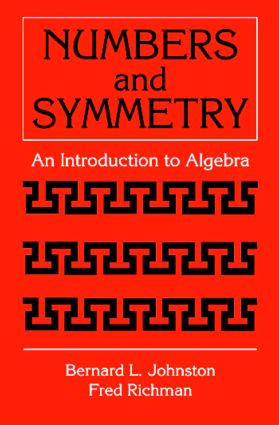# Numbers and Symmetry

## An Introduction to Algebra, 1st Edition

CRC Press

270 pages

##### Purchasing Options:\$ = USD
Paperback: 9780849303012
pub: 1997-01-07
SAVE ~\$14.19
\$70.95
\$56.76
x
Hardback: 9781138402096
pub: 2017-08-21
SAVE ~\$41.00
\$205.00
\$164.00
x

FREE Standard Shipping!

### Description

This textbook presents modern algebra from the ground up using numbers and symmetry. The idea of a ring and of a field are introduced in the context of concrete number systems. Groups arise from considering transformations of simple geometric objects. The analysis of symmetry provides the student with a visual introduction to the central algebraic notion of isomorphism.

Designed for a typical one-semester undergraduate course in modern algebra, it provides a gentle introduction to the subject by allowing students to see the ideas at work in accessible examples, rather than plunging them immediately into a sea of formalism. The student is involved at once with interesting algebraic structures, such as the Gaussian integers and the various rings of integers modulo n, and is encouraged to take the time to explore and become familiar with those structures.

In terms of classical algebraic structures, the text divides roughly into three parts:

Chapter 1. New Numbers

A Planeful of Integers, Z[i]

Circular Numbers, Zn

More Integers on the Number Line, Z [v2]

Notes

Chapter 2. The Division Algorithm

Rational Integers

Norms

Gaussian Numbers

Q (v2)

Polynomials

Notes

Chapter 3. The Euclidean Algorithm

Bézout's Equation

Relatively Prime Numbers

Gaussian Integers

Notes

Chapter 4. Units

Elementary Properties

Bézout's Equations

Wilson's Theorem

Orders of Elements: Fermat and Euler

Z [v2]

Notes

Chapter 5. Primes

Prime Numbers

Gaussian Primes

Z [v2]

Unique Factorization into Primes

Zn

Notes

Chapter 6. Symmetries

Symmetries of Figures in the Plane

Groups

The Cycle Structure of a Permutation

Cyclic Groups

The Alternating Groups

Notes

Chapter 7. Matrices

Symmetries and Coordinates

Two-by-Two Matrices

The Ring of Matrices M2(R)

Units

Complex Numbers and Quaternions

Notes

Chapter 8. Groups

Abstract Groups

Subgroups and Cosets

Isomorphism

The Group of Units of a Finite Field

Products of Groups

The Euclidean Groups E (1), E (2), and E (3)

Notes

Chapter 9. Wallpaper Patterns

One-Dimensional Patterns

Plane Lattices

Frieze Patterns

Space Groups

The 17 Plane Groups

Notes

Chapter 10. Fields

Polynomials Over a Field

Kronecker's Construction of Simple Field Extensions

Finite Fields

Notes

Chapter 11. Linear Algebra

Vector Spaces

Matrices

Row Space and Echelon Form

Inverses and Elementary Matrices

Determinants

Notes

Chapter 12. Error-Correcting Codes

Coding for Redundancy

Linear Codes

Parity-Check Matrices

Cyclic Codes

BCH Codes

CDs

Notes

Chapter 13. Appendix: Induction

Formulating the n-th Statement

The Domino Theory: Iteration

Formulating the Induction Statement

Squares

Templates

Recursion

Notes

Chapter 14. Appendix: The Usual Rules

Rings

Notes

Index

### Subject Categories

##### BISAC Subject Codes/Headings:
MAT000000
MATHEMATICS / General
MAT002000
MATHEMATICS / Algebra / General
MAT022000
MATHEMATICS / Number Theory